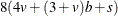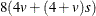# The SCORE Procedure

### Computational Resources

Let

 v = number of variables used in computing scores s = number of new score variables b = maximum number of new score variables in a BY group n = original input value

#### Memory

The array storage required is approximatelybytes. When you do not use BY processing, the array storage required is approximatelybytes.

#### Time

The time required to construct the scoring matrix is roughly proportional to, and the time needed to compute the scores is roughly proportional to.